# Gordon Growth Model

Updated June 9, 2021

## What Is the Gordon Growth Model?

The Gordon Growth Model (GGM) is a version of the dividend discount model (DDM). It is used to calculate the intrinsic value of a stock based on the net present value (NPV) of its future dividends.

## When Is the Gordon Growth Model Used?

Investors use the Gordon Growth Model to determine the relationship between valuation and return. However, the model is only accurate if certain conditions are met:

• The company has a stable business model.

• The company uses all of its free cash flow to pay dividends at regular intervals.

• The company’s dividends are expected to grow at a constant rate.

• The company’s other performance metrics (such as earnings) are also expected to grow at the same, stable rate as its dividends.

• The growth rate is lower than the investor’s required rate of return.

## Why Use the Gordon Growth Model?

By using the Gordon Growth method, investors can estimate the fair value of a stock to determine whether or not it is a viable investment. If (according to the appropriate inputs) the model presents a value higher than the current stock price, the stock is considered undervalued and is worth buying.

On the other hand, if the model presents a value that is lower than the current stock price, the stock is considered overvalued and is worth selling. Based on this value, investors can also make comparisons between other companies and industries.

## What Is the Gordon Growth Formula?

The formula for the Gordon Growth Model is as follows: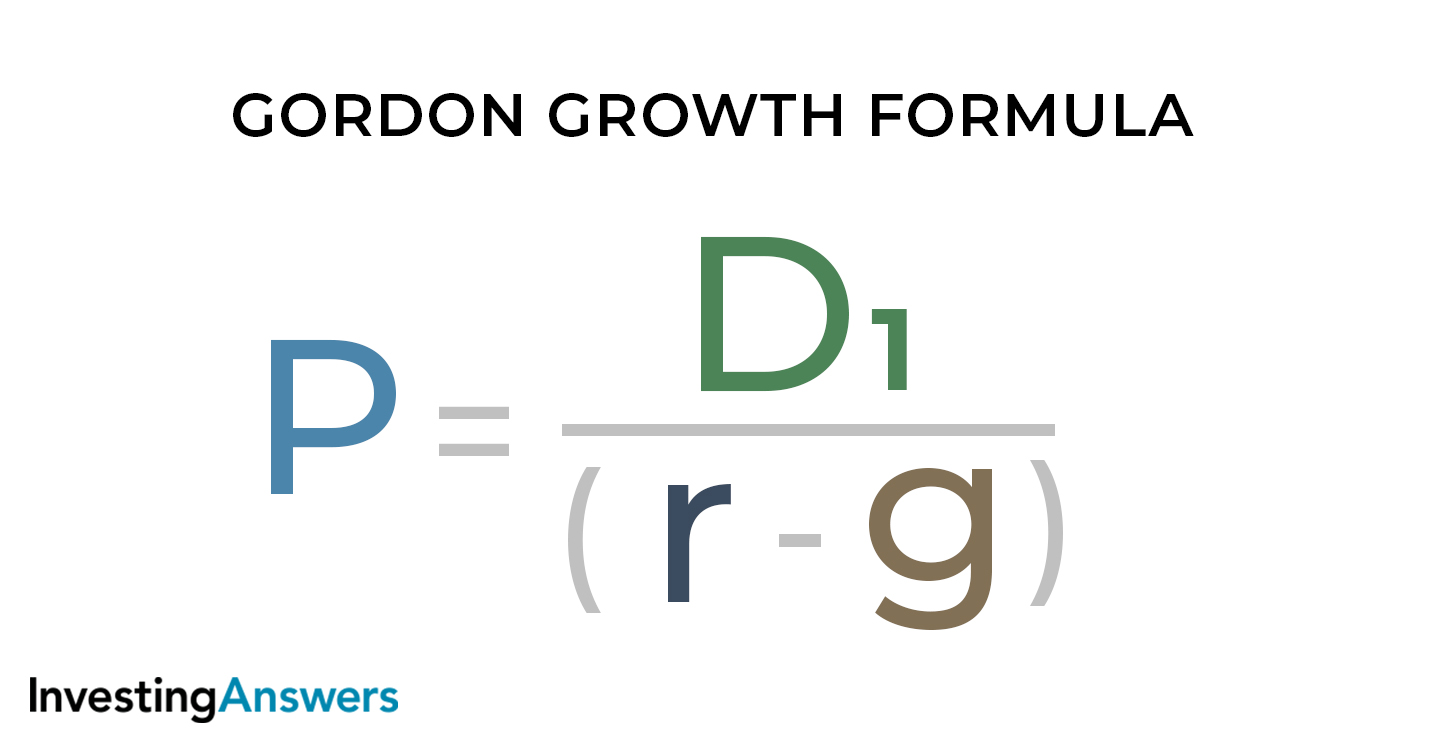Where:

P = Present value of stock
D1 = Value of next year's expected dividend per share
r = The investor's required rate of return (which can be found using the Capital Asset Pricing Model)
g = The expected dividend growth rate

### Expanded Gordon Growth Model

The Gordon Growth Model equates the present value of a company’s stock to the sum of an infinite series of discounted dividend payments. It is represented by the equation: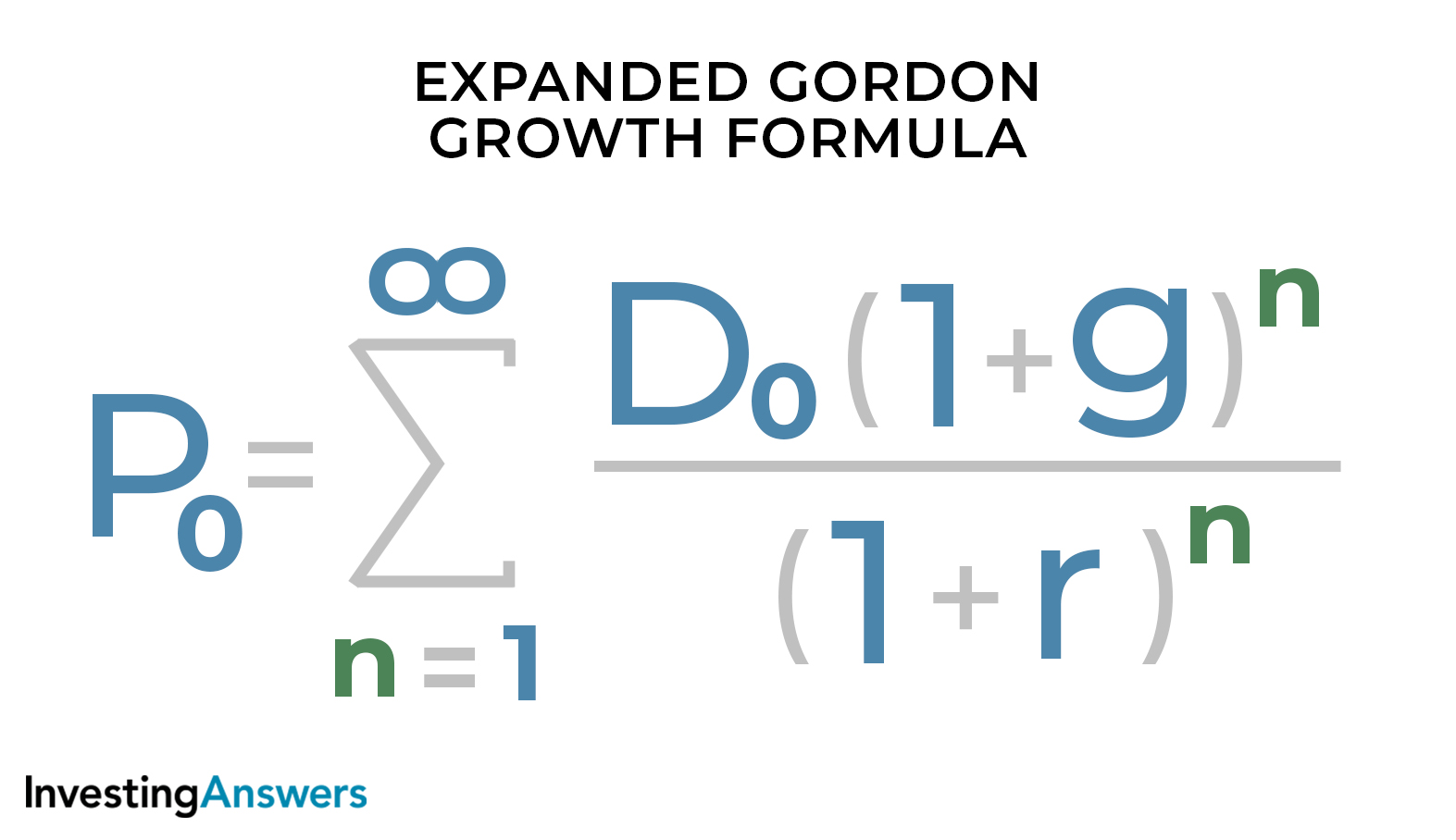Which is an infinite geometric series: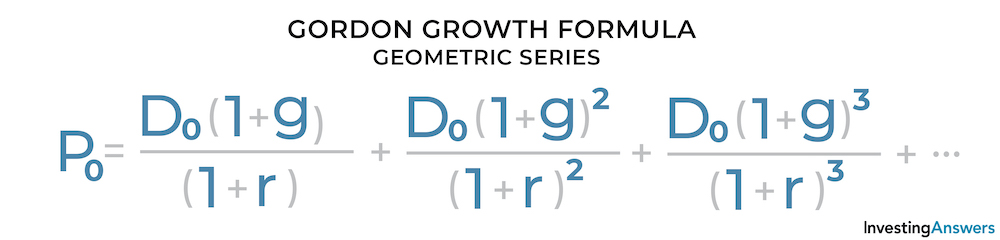Where:
P0 = Present value
n = Number of periods (years)
D0 = The initial value of each dividend payment
g = The expected dividend growth rate
r = Rate of return

### How to Derive the Gordon Growth Model

In order to derive the Gordon Growth Model, we’ll need to find the sum of the infinite geometric series using the following formula: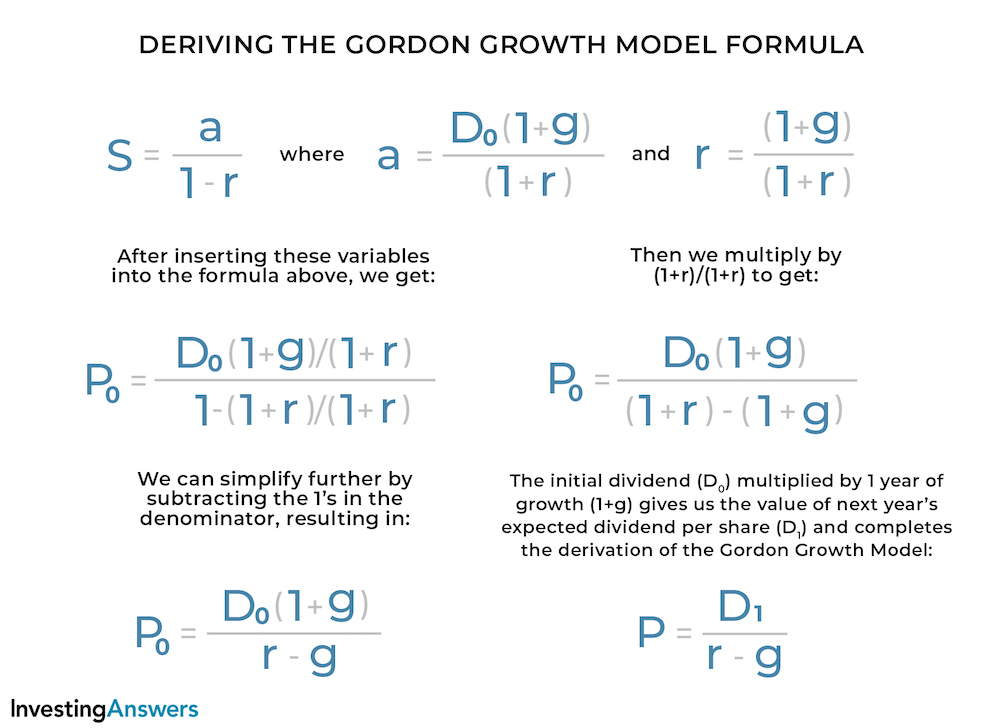## Gordon Growth Model Example

Suppose that Company A has a current stock price of \$100. It pays a \$1 dividend per share, which is expected to increase by 10% per year. An investor with a required rate of return of 5% wants to know the fair value of the stock. To determine whether to buy the stock, the investor can use the Gordon Growth Model: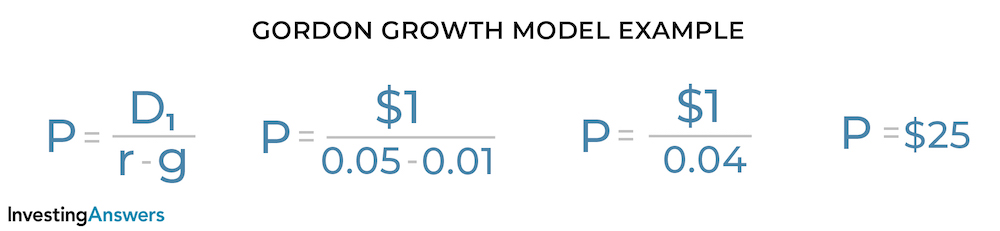In this situation, the fair value of the stock is \$25 (\$75 lower than the current trading price of \$100). This indicates that the stock is currently overvalued. The investor should not buy.

## Pros and Cons of the Gordon Growth Model

The Gordon Growth Model can be an effective way to analyze stocks, but – like most financial predictors – it has its pros and cons.

### Advantages of the Gordon Growth Model

Under the right conditions, the Gordon Growth Model is a useful tool for understanding the relationship between valuation and return. Here’s why:

#### Easy to Use

The model only requires three inputs and a simple calculation to solve for the value of a stock.

#### Inputs Are Easily Obtained

All of the inputs can be found on the company’s website or financial reports.

#### Can Help Make Comparisons

This model allows investors, analysts, and companies to compare stocks of different companies and industries.

### Disadvantages of the Gordon Growth Model

There are some drawbacks to the Gordon Growth Model:

#### Dividend Growth Rate Must Be Constant

GGM requires that the dividend growth rate is constant (which is not always an accurate assumption in real life). Constant dividend growth rates are more common among mature companies in mature industries due to their stability. On the other hand, constant growth rates are less common among newer companies and industries due to potential fluctuations and volatility as they develop.

#### Required Rate of Return Must Be Higher Than Growth Rate

The required rate of return/cost of equity must be higher than the dividend growth rate. Otherwise, the model will provide a negative value.

#### Ignores External Factors

The model ignores a number of important external factors that can influence the value of a company and/or its stock.

#### Only Works For Companies That Pay Dividends

This model only applies to companies that pay dividends. However, not all companies pay dividends, so this limits the number of stocks and companies that the model can be used to analyze.

In order to increase growth potential, newer companies may reinvest their earnings instead of paying dividends. On the other hand, more mature companies may pay dividends to demonstrate strength, stability, and expectations of future earnings.

All of our content is verified for accuracy by Rachel Siegel, CFA and our team of certified financial experts. We pride ourselves on quality, research, and transparency, and we value your feedback. Below you'll find answers to some of the most common reader questions about Gordon Growth Model.

### Can You Calculate Weighted Average Cost of Capital (WACC) Using the Gordon Growth Model?

Yes. The Gordon Growth Model can be rearranged to solve for the cost of capital, as shown below...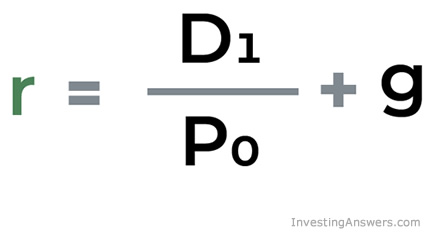### Can Gordon Growth Model Handle Negative Growth Rate?

Yes. A negative growth rate will increase the value of the denominator, resulting in a lower present value.

### Is the Gordon Growth Model Accurate?

Provided that all conditions are met, yes, the Gordon Growth Model is accurate. However, one of the major limitations of this model is that growth is rarely constant in real market conditions. And if growth is not constant, the model is not accurate.

### Who Invented the Gordon Growth Model?

The Gordon Growth Model was named after Myron J. Gordon of the University of Toronto. It was originally published by Gordon and other scholars in 1956 but was heavily based on a 1938 book called "The Theory of Investment Value" by John Burr Williams.

Rachel Siegel, CFA - 141Rachel Siegel, CFACFA Charterholder

Chartered Financial Analyst

Rachel Siegel, CFA is one of the nation's leading experts at ensuring the accuracy of financial and economic text.  Her prestigious background includes over 10 years creating professional financial certification exams and another 20 years of college-level teaching.